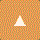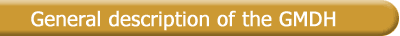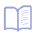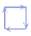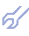There were published many papers and books devoted to the Group Method of Data Handling theory and its applications. The GMDH can be considered as further propagation of inductive self-organizing methods to the solution of more complex practical problems. It solves the problem of how to handle data samples of observations. The goal is to get mathematical model of the object (the problem of identification and pattern recognition) or to describe the processes, which will take place at object in the future (the problem of process forecasting). GMDH solves, by sorting-out procedure, the multidimensional problem of model optimization: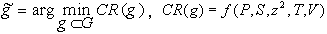where: G - set of considered models; CR is an external criterion of model g quality from this set; P - number of variables set; S - model complexity; z2 - noise dispersion; T - number of data sample transformation; V - type of reference function. For definite reference function, each set of variables corresponds to definite model structure P = S. Problem transforms to much simpler one-dimensionalwhen z2= const, T = const, and V = const. Method is based on the sorting-out procedure, i.e. consequent testing of models, chosen from set of models-candidates in accordance with the given criterion. Most of GMDH algorithms, use the polynomial reference functions. General connection between input and output variables can be expressed by Volterra functional series, discrete analogue of which is Kolmogorov-Gabor polynomial:where X(x1,x2,...,xM,); - input variables vector; A(a1,a2,...,aM,); - vector of coefficients or weights; Components of the input vector X can be independent variables, functional forms or finite difference terms. Other non-linear reference functions, such as difference, probabilistic, harmonic, logistic can also be used. The method allows to find simultaneously the structure of model and the dependence of modelled system output on the values of most significant inputs of the system. long-term forecasting [3,18]; short-term forecasting of processes and events ; identification of physical regularities; approximation of multivariate processes; physical fields extrapolation ; data samples clusterization ; pattern recognition in the case of continuous-valued or discrete variables; diagnostics and recognition by probabilistic sorting-out algorithms ; decision support after "what-if" scenario and vector process normative forecasting ; modelless processes forecasting using analogues complexing ; self-organization of twice-multilayered neuronets with active neurons [9,10]. In  were obtained the theoretical grounds of GMDH effectiveness as adequate method of robust forecasting models construction, essence of which consists of automatically generation of models in given class by sequential selection of the best of them by criteria, which implicitly by sample dividing take into account the level of indeterminacy. Since 1968 a big number of GMDH technique implementations for modeling of economic, ecological, environmental, medical, physical and military objects were done in several countries. Some outdated approaches are used in USA by Barron Associates Co. in "ASPN-II", AbTech Corp. in "ModelQuest" (AIM), by Ward Systems Group, Inc. in "NeuroShell2", and DeltaDesign Software in "SelfOrganize!" commercial software tools. Self-organizing modeling is based on statistical learning networks, which are networks of mathematical functions that capture complex, non-linear relationships in a compact and rapidly executable form. Such networks subdivide a problem into manageable pieces or nodes and then automatically apply advanced regression techniques to solve each of these simpler problems. Special GMDH peculiarities The main peculiarity of GMDH algorithms is that, when it uses continuous data with noise, it selects as optimal simplified non-physical model. Only for accurate and discrete data the algorithms point out so-called physical model - the most simple optimal, from all unbiased models. It is proved the convergence of multilayered GMDH algorithms  and it is proved that shortened non-physical model is better than full physical model on error criterion (for noisy and continuous data for prediction and approximation solving, more simplified Shennon's non-physical models become more accurate ). It can be noted, that this conclusion has place in model selection on the basis of models entropy maximization (Akaike approach), in average risk minimizing (Vapnik approach) and in another modern approaches. The only way to get non-physical models is to use sorting-out GMDH algorithms. Usage of sorting-out procedure guarantees selection of the best optimal model from all possible. Regularity of optimal structure of forecasting models change in dependence on general indexes of data indeterminacy (noise level, data sample length, design of experiment, number of informational variables) was shown in [24,25,27]. The special peculiarities of GMDH are following : External supplement:Following S.Beer work , only the external criteria, calculated on new independent information,can produce the minimum of sorting-out characteristic. Because of this data sampling is divided into parts for model construction and evaluation. Freedom of choice:Following D.Gabor work , in multilayered GMDH algorithms are to be conveyed from one layer to the next layer not all but F best results of choice to provide "freedom of choice" The rule of layers complication:Partial descriptions (forms of a mathematical description for iteration) should be simple, without quadratic members in them; Additional model definition:In cases, when the choice of optimal physical model is difficult, because of noise level or oscillations of criterion minima characteristic, auxiliary discriminating criterion is used . The choice of the main criterion and constrains of sorting-out procedure is the main heuristic of GMDH; All algorithms have multilayered structure and parallel computing can be implemented for their realization. All questions that arise about type of algorithm, criterion, variables set choice should be solved by minimal value of external criterion. The main criteria proposed are: cross-validation PRR(s), regularity AR(s) and balance of variables criterion BL(s). Estimation of their effectiveness (investigation of noise immunity, optimality and adequateness) and their comparison with another criteria was done in detail in [24,25,26,15]. The conditions, under which GMDH algorithm produces the minimum of characteristics are following: criterion of model choice is to be external, based on additional fresh information, which was not used for model construction; the data sample is not to be too long. Such data sample produce the same model of usual regression analysis for exact data; when difference type balance criterion BL(s) is used, small noise is necessary or the variables in the data sample should not be exactly measured . Difference of the GMDH algorithms from another algorithms of structural identification and best regression selection algorithms consists of three main peculiarities: usage of external criteria, which are based on data sample dividing and are adequate to problem of forecasting models construction, by decreasing of requirements to volume of initial information; more diversity of structure generators: usage like in regression algorithms of the ways of full or reduced sorting of structure variants and of original multilayered (iteration) procedures; better level of automatization: there are needed to enter initial data sample and type of external criterion only; automatic adaptation of optimal model complexity and external criteria to level of noises or statistical violations - effect of noiseimmunity cause robustness of the approach; implementation of principle of inconclusive decisions in process of gradual models complication.